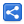# Java 9 Collection Changes

[Last Updated: Oct 11, 2017]

Following is a quick walk-through of Collection changes in Java 9.

## Set

A group of new factory methods provide a convenient way to create an instance of immutable java.util.Set.

` Set<Integer> integers = Set.of(2, 6, 7, 10); System.out.println(integers);`
``` [2, 10, 6, 7]
```

There are multiple such overloaded methods.

``` of()//empty set
of(E)
of(E, E)
of(E, E, E)
//more ......
of(E, E, E, E, E, E, E, E, E, E )// up to 10 elements
of(E...)//with varargs```

## List

Similar to Set, List also has new factory methods (same number of overloaded methods) to create an immutable instance:

` List<Integer> integers = List.of(2, 6, 7, 10); System.out.println(integers);`
``` [2, 6, 7, 10]
```

## Map

Map also has new factory methods to create immutable instances. These methods accept key/value pairs as arguments which should be in alternate positions.

` Map<Integer, String> map = Map.of(2, "two", 6, "six"); System.out.println(map);`
``` {2=two, 6=six}
```
Similar to set/list, we have 11 such overloaded methods:
``` of()//empty
of(K, V)
of(K, V, K, V)
of(K, V, K, V, K, V)
//more ....
of(K, V, K, V, K, V, K, ...)//10 pairs
```

### Map.ofEntries() and Map.entry() methods

The factory method `Map.ofEntries` accepts the Map.Entry as varargs. There is another related new static method `Map.entry(K, V)` to create an instance of Entry.

` Map<Integer, String> map = Map.ofEntries(Map.entry(2, "two"), Map.entry(4, "four")); System.out.println(map);`
``` {2=two, 4=four}
```

## Arrays

### Arrays.mismatch() methods

These new methods are used to find the index of first mismatch between two arrays. For example following finds the first mismatch between two arrays of integers.

` int[] ints1 = {1, 3, 5, 7, 9}; int[] ints2 = {1, 3, 5, 6, 7}; int i = Arrays.mismatch(ints1, ints2); System.out.println(i);`
``` 3
```

This method will return -1 if there are no mismatch. Also matching is performed within a length range of the smaller array.

We have similar overloaded methods for other types of arrays (primitives, objects and generic T type)

Another group of such methods accepts fromIndex and toIndex parameters (for each arrays) to find the relative mismatch index. For example:

` int mismatch(int[] a, int aFromIndex, int aToIndex, int[] b, int bFromIndex, int bToIndex)`
` int[] arrayA = {-2, 1, 3, 5, 7, 9}; int[] arrayB = {-1, 0, 1, 3, 5, 7, 10}; int j = Arrays.mismatch(arrayA, 1, arrayA.length, arrayB, 2, arrayB.length); System.out.println(j);`
``` 4
```
Above is equivalent to finding the mismatch in the following:
` int[] arrayC = {1, 3, 5, 7, 9}; int[] arrayD = {1, 3, 5, 7, 10}; int k = Arrays.mismatch(arrayC, arrayD); System.out.println(k);`
``` 4
```

### Arrays.compare() methods

These new methods compare the elements of the two provided arrays. Following is one of such methods:

` int compare(int[] a, int[] b) `

There are overloaded methods for other primitives and object type (with generics T signature).

These methods inter-compare each element of the arrays in lexicographical (alphabetical) order.

Example:

` String[] stringsA = {"one", "two"}; String[] stringsB = {"four", "three"}; int i = Arrays.compare(stringsA, stringsB); System.out.println(i);  //can be used to sort collection of arrays List<String[]> list = Arrays.asList(stringsA, stringsB); System.out.print("before: "); list.forEach(a -> System.out.print(Arrays.toString(a))); Collections.sort(list, Arrays::compare);//java 8 method reference System.out.print("\nafter:  "); list.forEach(a -> System.out.print(Arrays.toString(a)));`
``` 9
before: [one, two][four, three]
after:  [four, three][one, two]
```

Following are the other variants of compare methods:

• With fromIndex/toIndex parameters which are used for relative position comparision (similar to what we saw in mismatch() methods above).
• With Comparator parameter, which can be used for customized comparing (instead of lexicographical comparing)
• `Arrays.compareUnsigned()`: these method (only for numeric arrays) compare each element as unsigned.

### Arrays.equals() methods

More overloaded methods have been added to the `Arrays.equals()` group. The new methods additionally takes fromIndex and toIndex parameters for the two provided arrays. These methods check equality of the two arrays based on their relative index positions.

Following is one of those methods:

` boolean equals(Object[] a, int aFromIndex, int aToIndex, Object[] b, int bFromIndex, int bToIndex)    `

Example:

` String[] sa = {"d", "e", "f", "g", "h"}; String[] sb = {"a", "b", "c", "d", "e", "f"}; boolean b = Arrays.equals(sa, 0, 2, sb, 3, 5); System.out.println(b);`
``` true
```

## Enumeration

### Enumeration.asIterator()

This new method returns an instance of `java.util.Iterator` which can be used to traverse the remaining elements covered by this enumeration.

` Vector<Integer> aVector = new Vector<>(Arrays.asList(1, 2, 4, 5, 6)); Enumeration<Integer> enumeration = aVector.elements(); Iterator<Integer> iterator = enumeration.asIterator(); iterator.forEachRemaining(System.out::println);`
``` 1
2
4
5
6
```

 Share